# RD Sharma Solutions for Class 9 Maths Chapter 15 Area of Parallelograms and Triangles Exercise 15.3

Get free RD Sharma Solutions for Class 9 Maths Chapter 15 exercise 15.3 here. In this exercise, students will learn figures on the same base and between the same parallels, mainly parallelograms and triangles. The step by step solution in RD Sharma Solution for Class 9 Mathematics offers invaluable help while preparing for exams.

## Download PDF of RD Sharma Solutions for Class 9 Maths Chapter 15 Area of Parallelograms and Triangles Exercise 15.3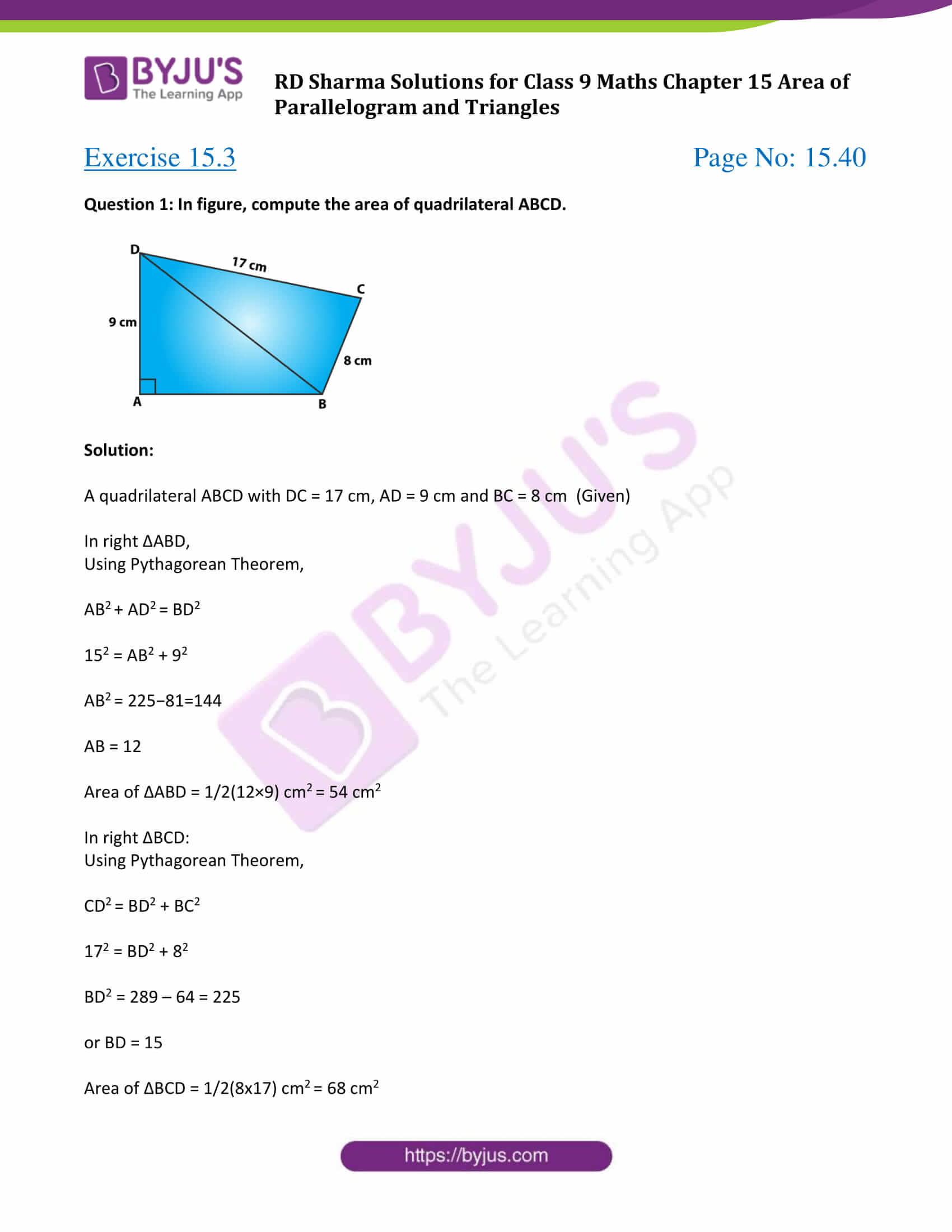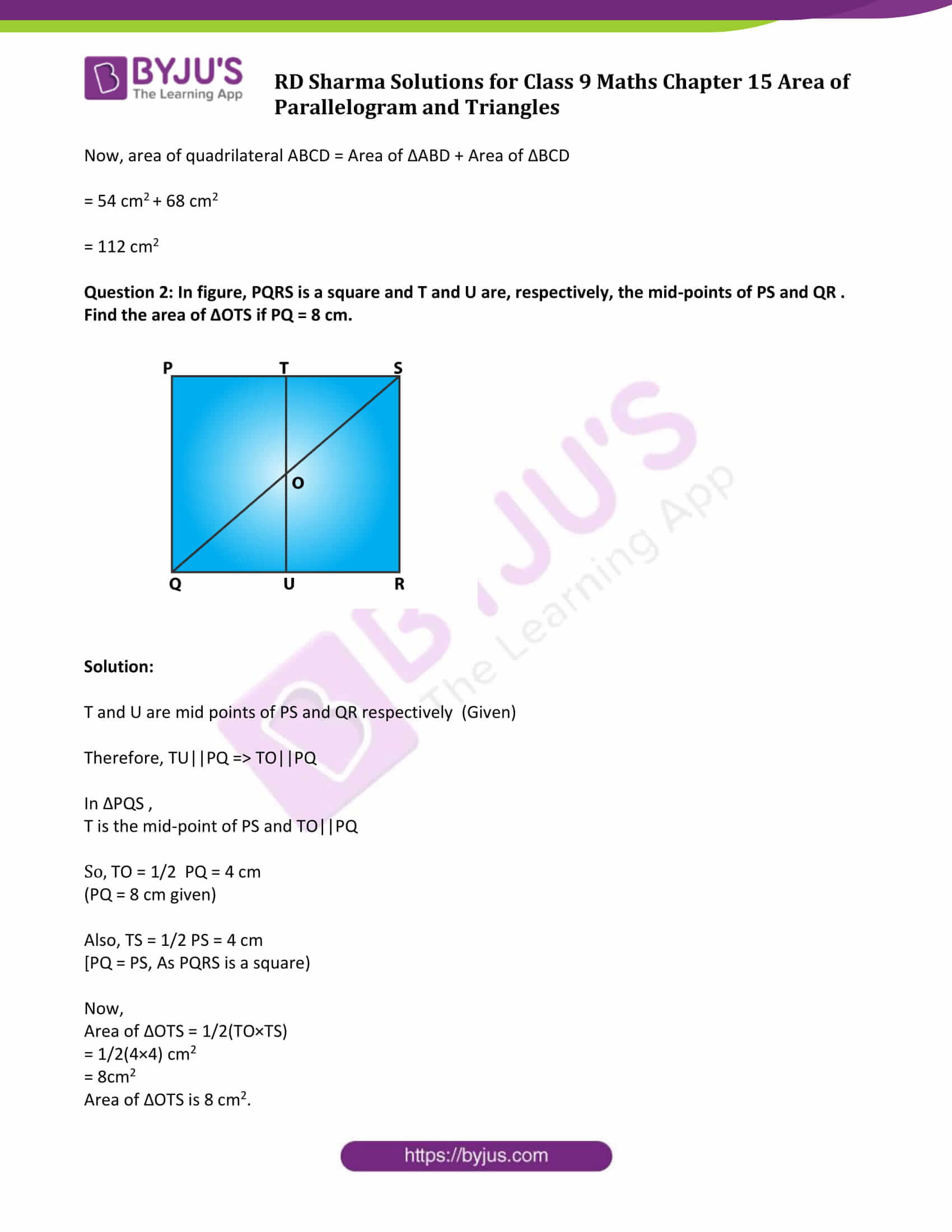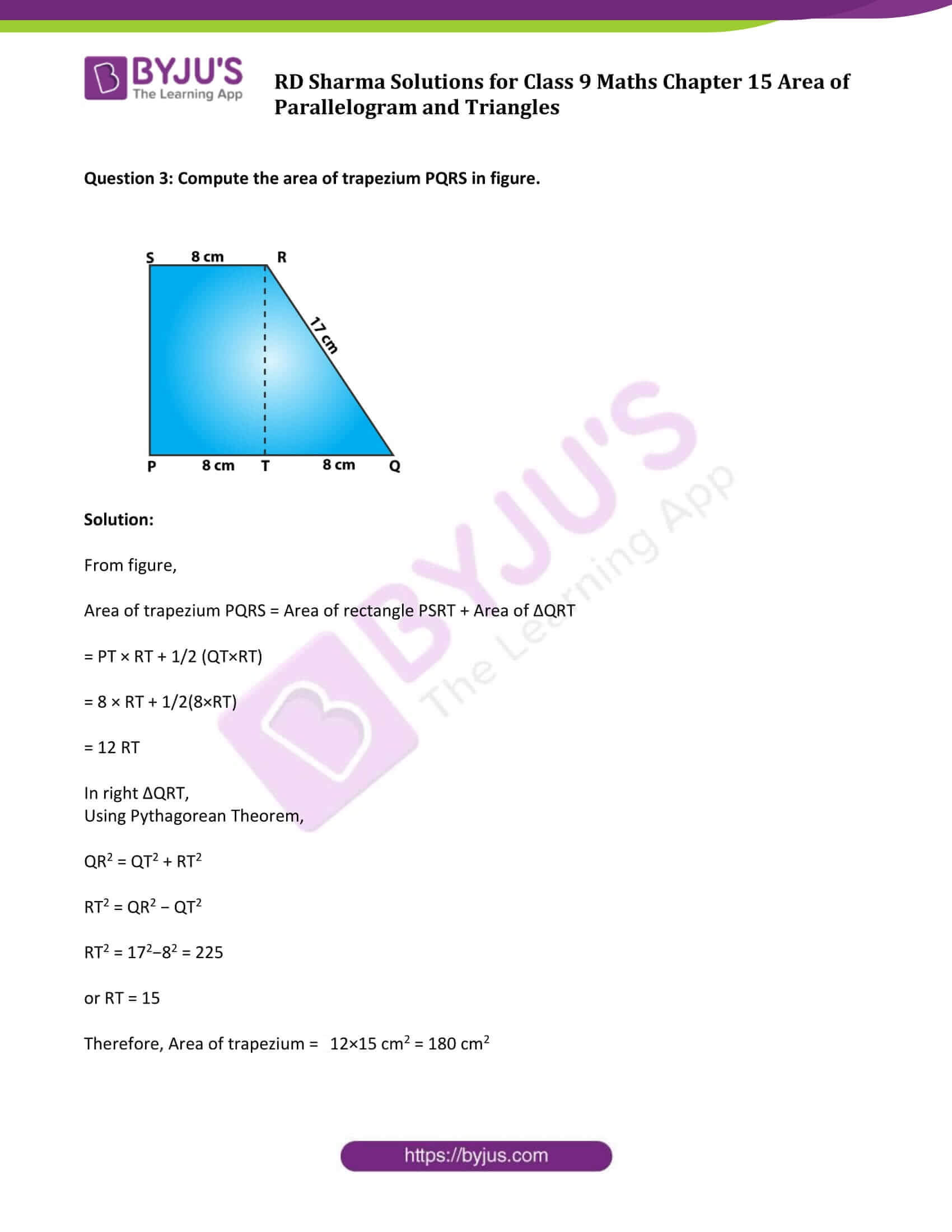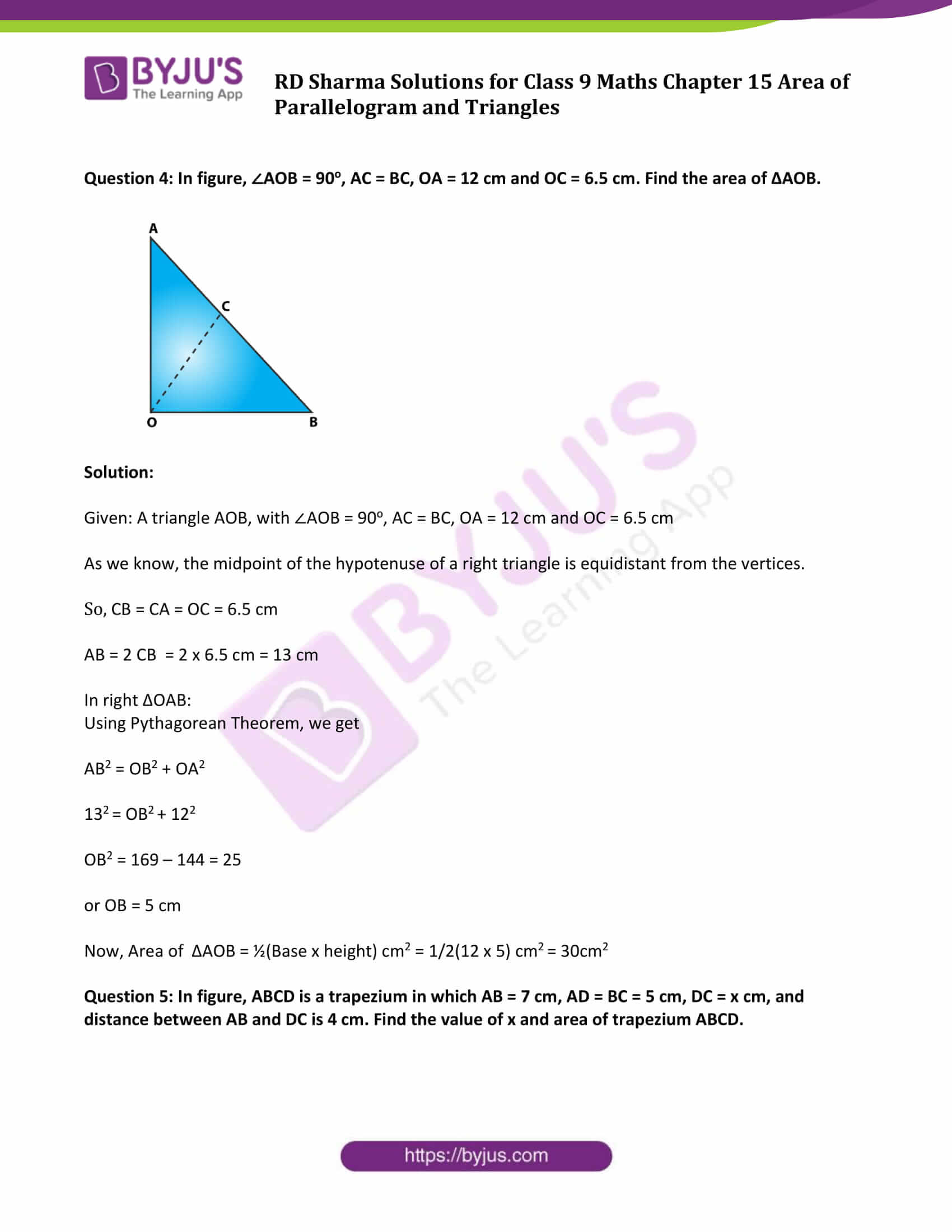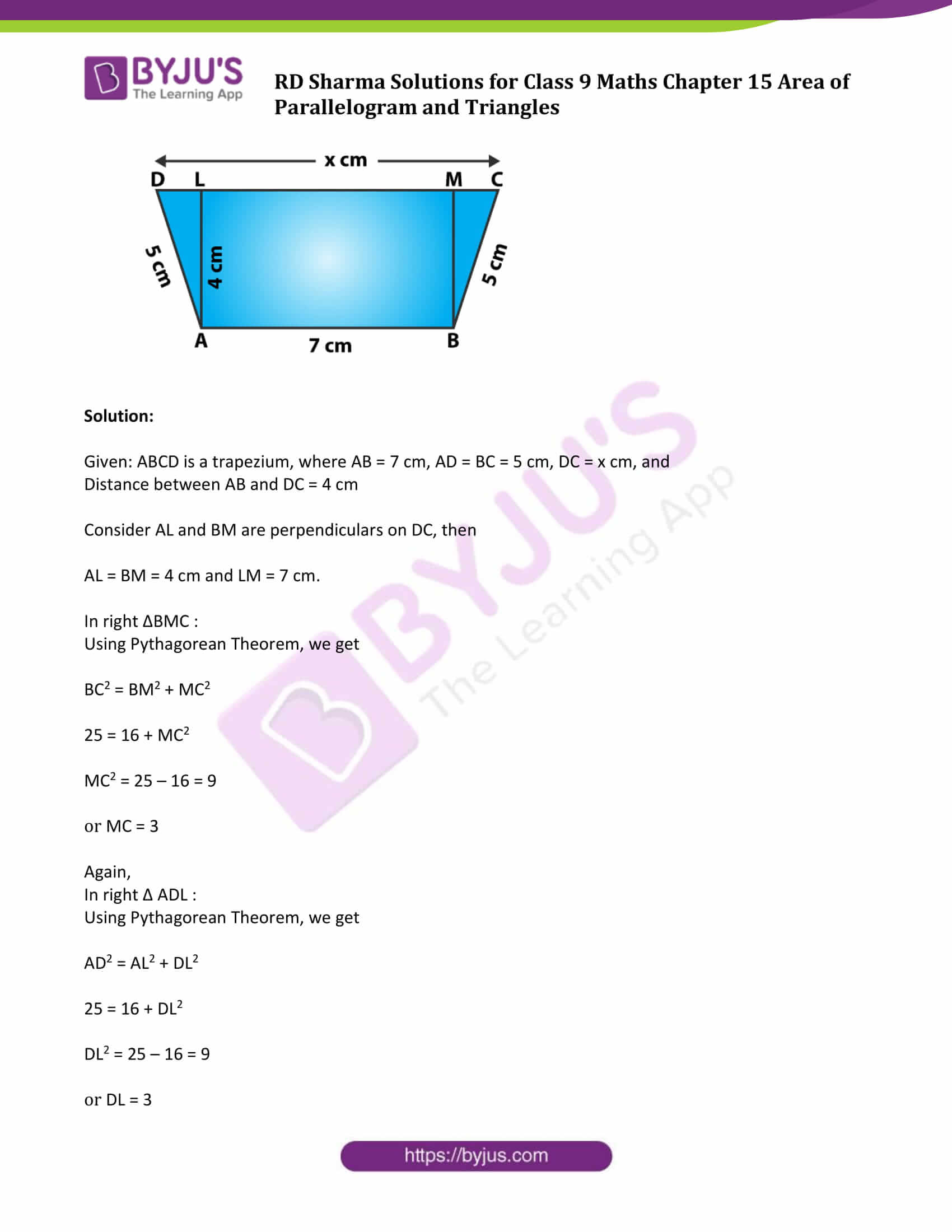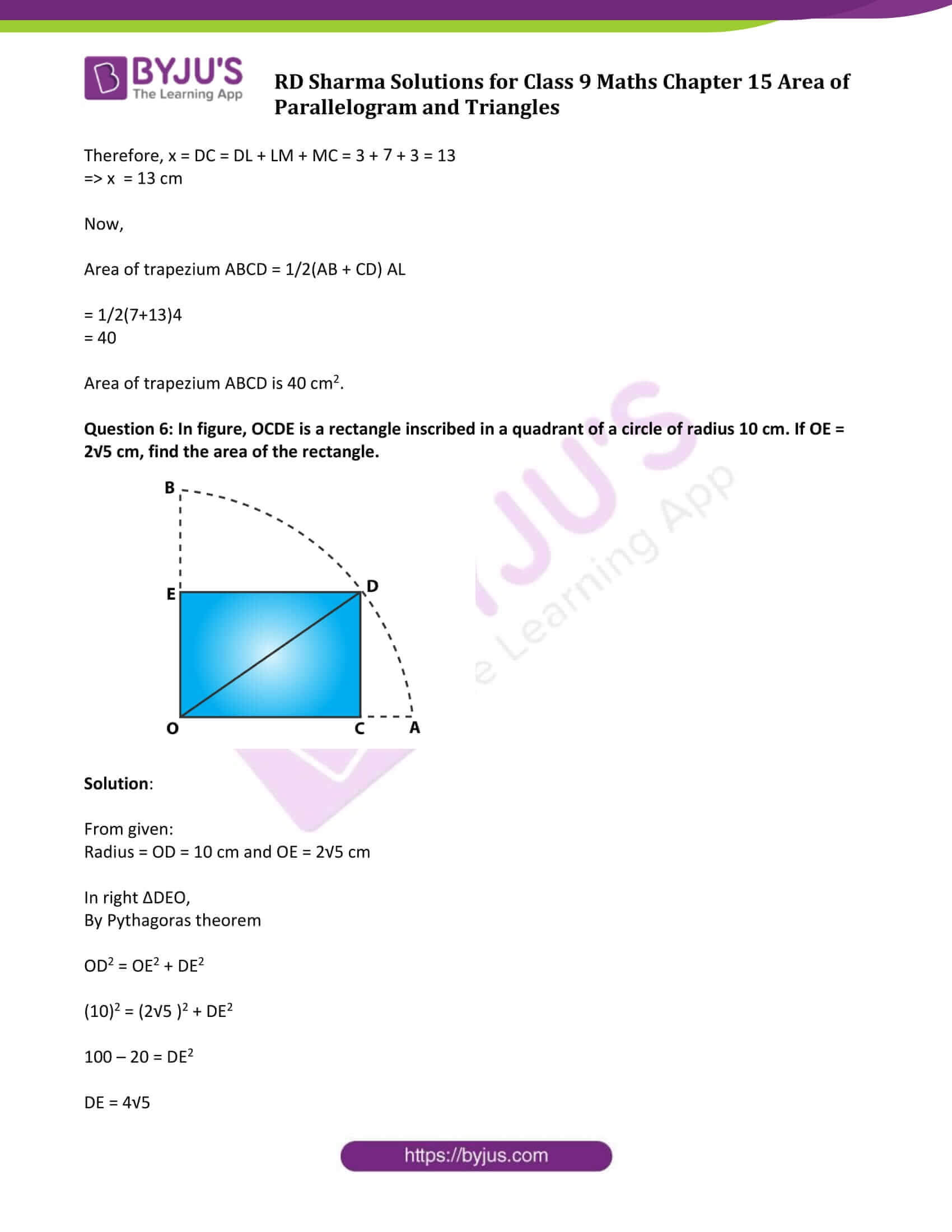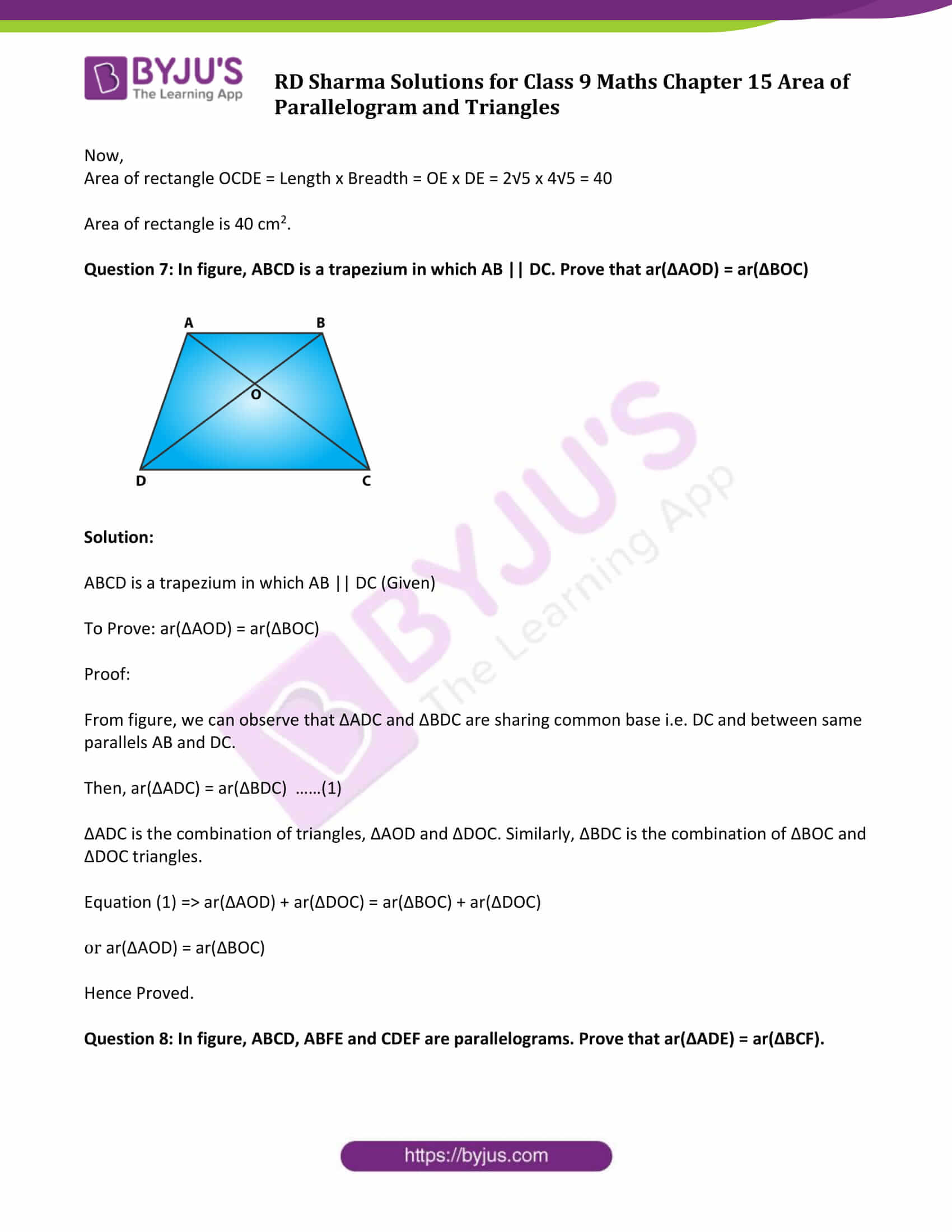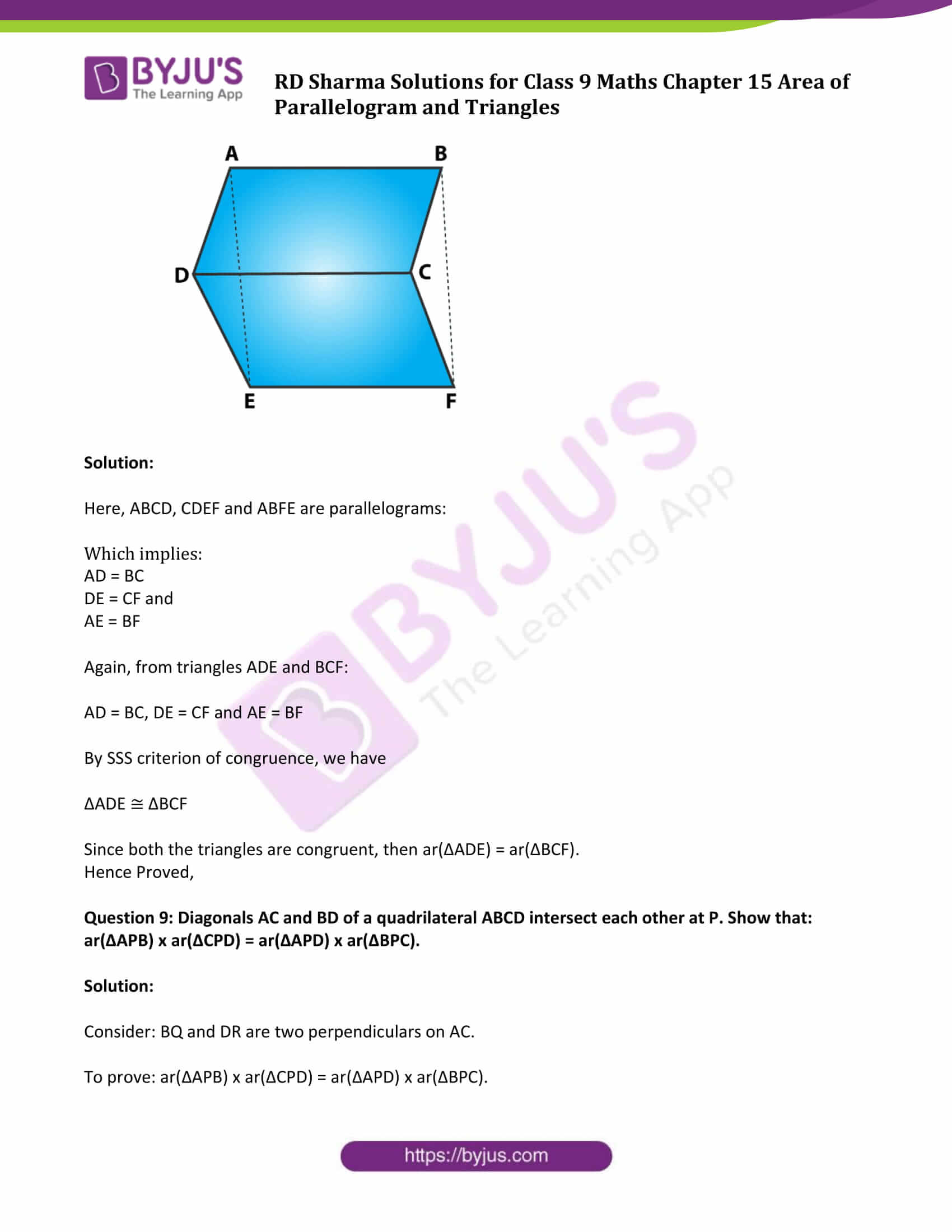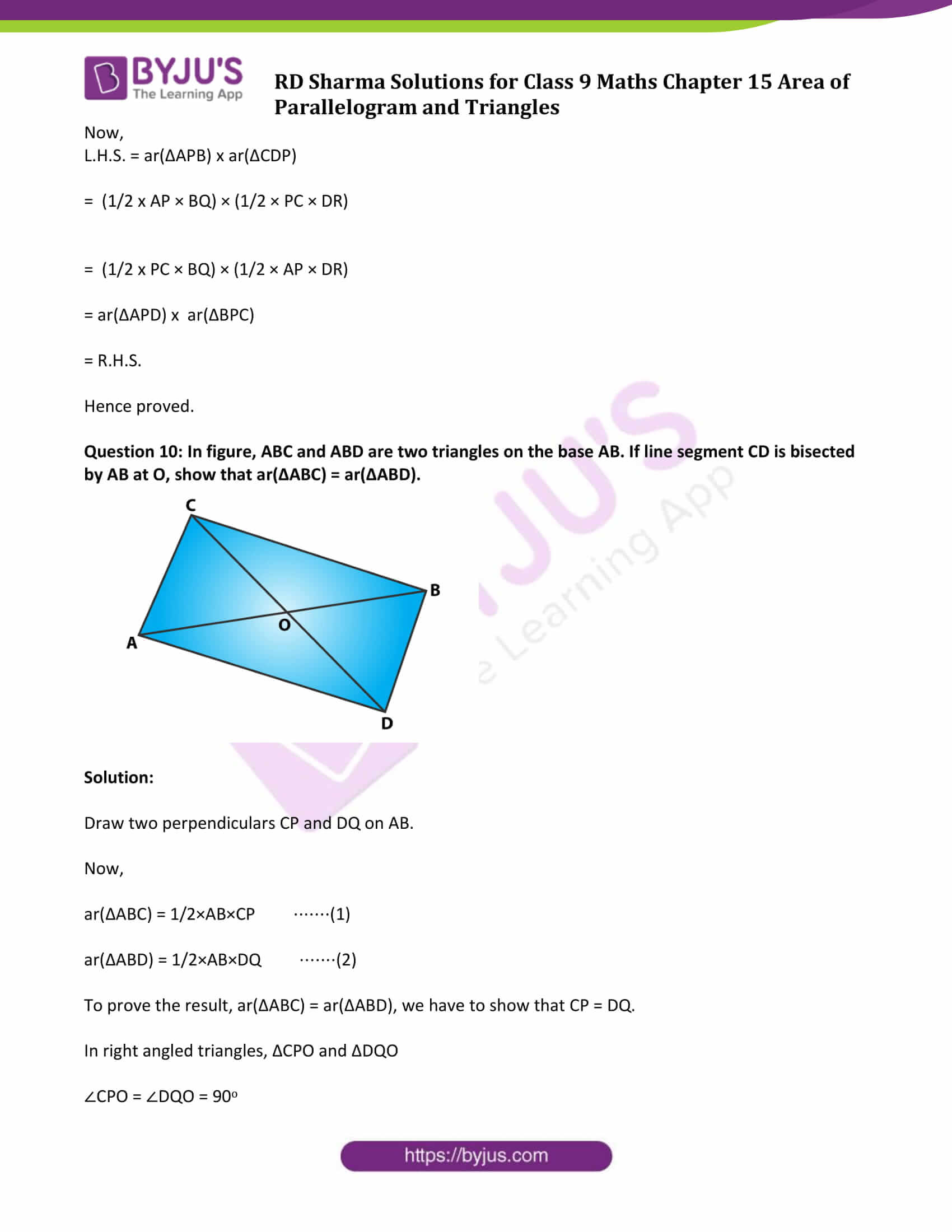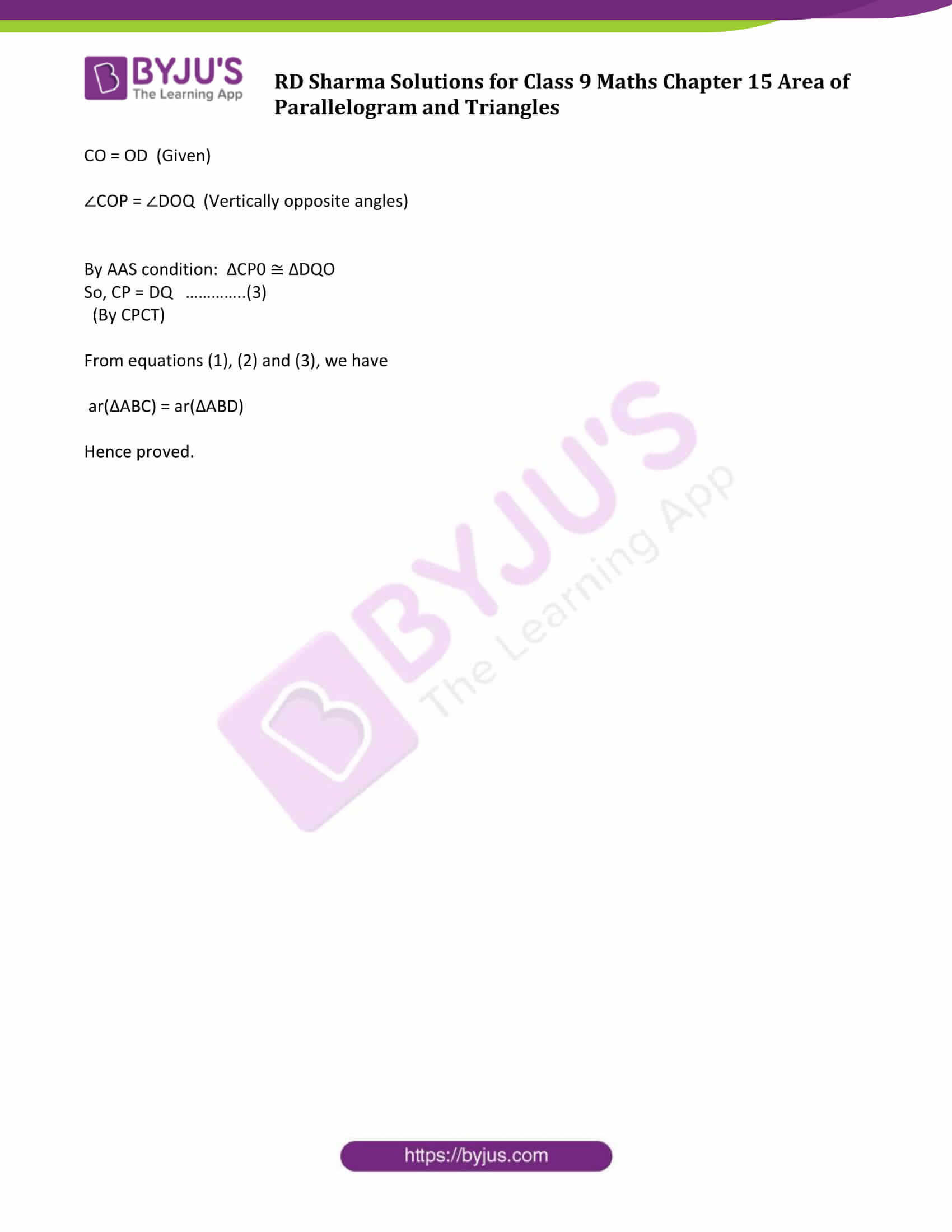### Access Answers to Maths RD Sharma Solutions for Class 9 Chapter 15 Area of Parallelograms and Triangles Exercise 15.3 Page number 15.40

Question 1: In figure, compute the area of quadrilateral ABCD.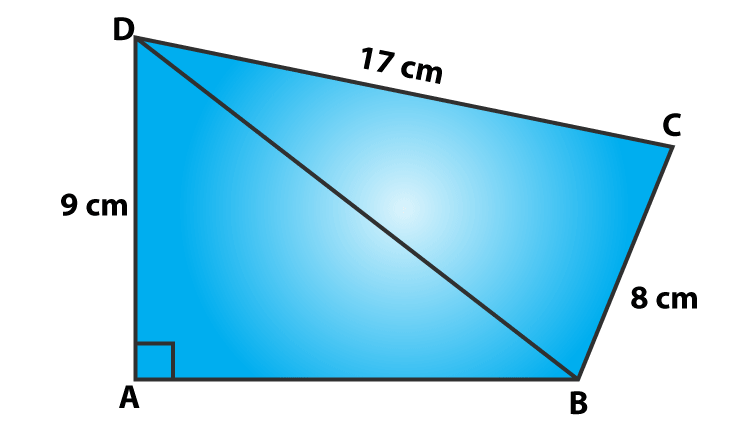Solution:

A quadrilateral ABCD with DC = 17 cm, AD = 9 cm and BC = 8 cm (Given)

In right ΔABD,

Using Pythagorean Theorem,

152 = AB2 + 92

AB2 = 225−81=144

AB = 12

Area of ΔABD = 1/2(12×9) cm2 = 54 cm2

In right ΔBCD:

Using Pythagorean Theorem,

CD2 = BD2 + BC2

172 = BD2 + 82

BD2 = 289 – 64 = 225

or BD = 15

Area of ΔBCD = 1/2(8×17) cm2 = 68 cm2

Now, area of quadrilateral ABCD = Area of ΔABD + Area of ΔBCD

= 54 cm2 + 68 cm2

= 112 cm2

Question 2: In figure, PQRS is a square and T and U are, respectively, the mid-points of PS and QR . Find the area of ΔOTS if PQ = 8 cm.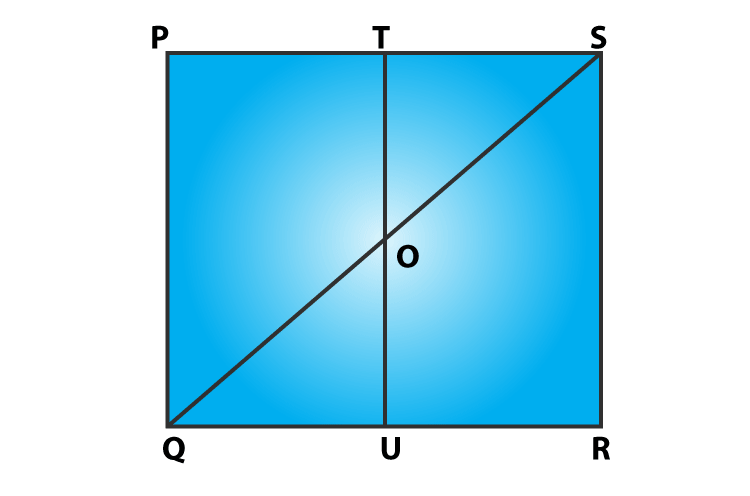Solution:

T and U are mid points of PS and QR respectively (Given)

Therefore, TU||PQ => TO||PQ

In ΔPQS ,

T is the mid-point of PS and TO||PQ

So, TO = 1/2 PQ = 4 cm

(PQ = 8 cm given)

Also, TS = 1/2 PS = 4 cm

[PQ = PS, As PQRS is a square)

Now,

Area of ΔOTS = 1/2(TO×TS)

= 1/2(4×4) cm2

= 8cm2

Area of ΔOTS is 8 cm2.

Question 3: Compute the area of trapezium PQRS in figure.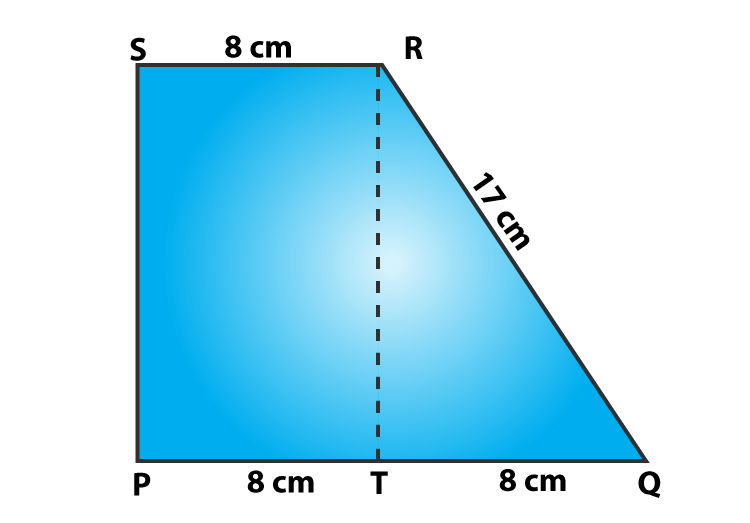Solution:

From figure,

Area of trapezium PQRS = Area of rectangle PSRT + Area of ΔQRT

= PT × RT + 1/2 (QT×RT)

= 8 × RT + 1/2(8×RT)

= 12 RT

In right ΔQRT,

Using Pythagorean Theorem,

QR2 = QT2 + RT2

RT2 = QR2 − QT2

RT2 = 172−82 = 225

or RT = 15

Therefore, Area of trapezium = 12×15 cm2 = 180 cm2

Question 4: In figure, ∠AOB = 90o, AC = BC, OA = 12 cm and OC = 6.5 cm. Find the area of ΔAOB.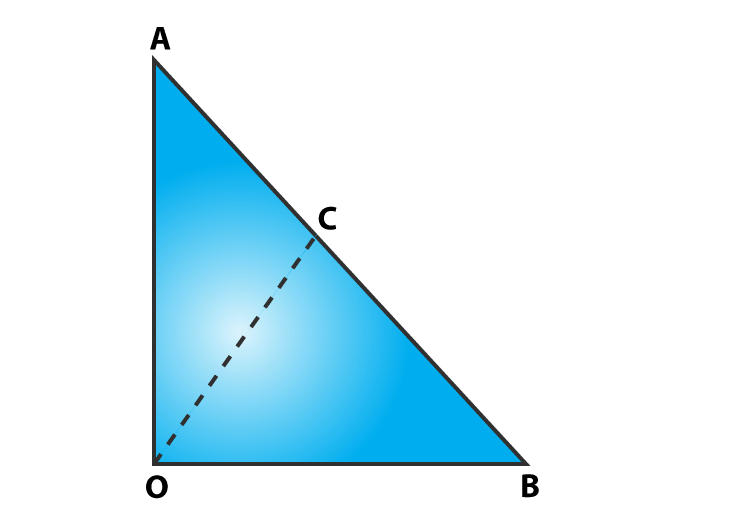Solution:

Given: A triangle AOB, with ∠AOB = 90o, AC = BC, OA = 12 cm and OC = 6.5 cm

As we know, the midpoint of the hypotenuse of a right triangle is equidistant from the vertices.

So, CB = CA = OC = 6.5 cm

AB = 2 CB = 2 x 6.5 cm = 13 cm

In right ΔOAB:

Using Pythagorean Theorem, we get

AB2 = OB2 + OA2

132 = OB2 + 122

OB2 = 169 – 144 = 25

or OB = 5 cm

Now, Area of ΔAOB = ½(Base x height) cm2 = 1/2(12 x 5) cm2 = 30cm2

Question 5: In figure, ABCD is a trapezium in which AB = 7 cm, AD = BC = 5 cm, DC = x cm, and distance between AB and DC is 4 cm. Find the value of x and area of trapezium ABCD.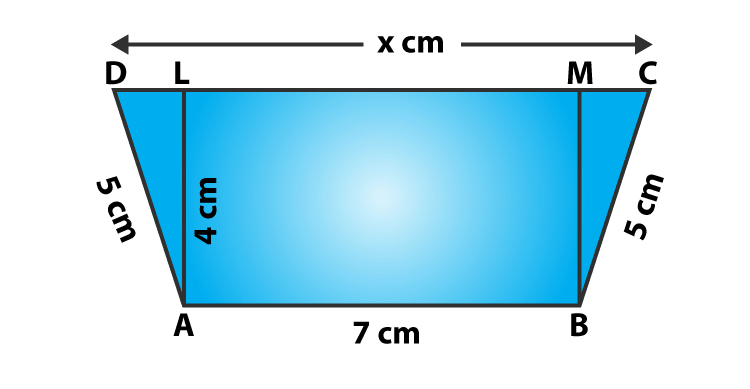Solution:

Given: ABCD is a trapezium, where AB = 7 cm, AD = BC = 5 cm, DC = x cm, and

Distance between AB and DC = 4 cm

Consider AL and BM are perpendiculars on DC, then

AL = BM = 4 cm and LM = 7 cm.

In right ΔBMC :

Using Pythagorean Theorem, we get

BC2 = BM2 + MC2

25 = 16 + MC2

MC2 = 25 – 16 = 9

or MC = 3

Again,

Using Pythagorean Theorem, we get

25 = 16 + DL2

DL2 = 25 – 16 = 9

or DL = 3

Therefore, x = DC = DL + LM + MC = 3 + 7 + 3 = 13

=> x = 13 cm

Now,

Area of trapezium ABCD = 1/2(AB + CD) AL

= 1/2(7+13)4

= 40

Area of trapezium ABCD is 40 cm2.

Question 6: In figure, OCDE is a rectangle inscribed in a quadrant of a circle of radius 10 cm. If OE = 2√5 cm, find the area of the rectangle.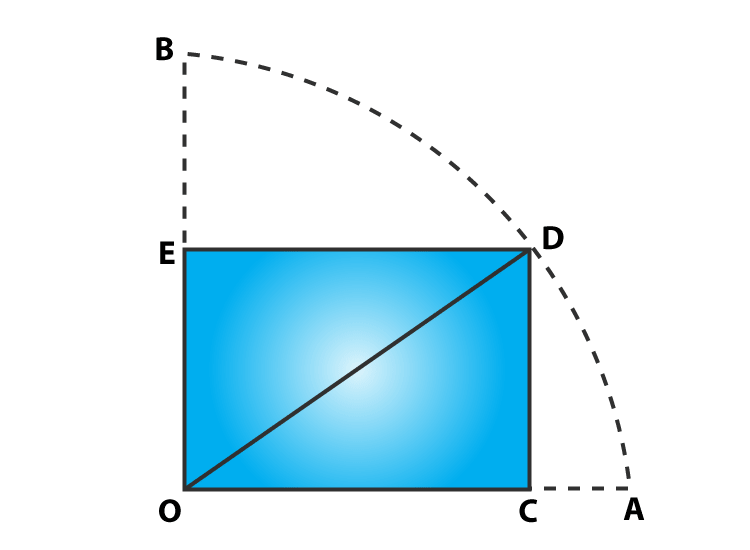Solution:

From given:

Radius = OD = 10 cm and OE = 2√5 cm

In right ΔDEO,

By Pythagoras theorem

OD2 = OE2 + DE2

(10)2 = (2√5 )2 + DE2

100 – 20 = DE2

DE = 4√5

Now,

Area of rectangle OCDE = Length x Breadth = OE x DE = 2√5 x 4√5 = 40

Area of rectangle is 40 cm2.

Question 7: In figure, ABCD is a trapezium in which AB || DC. Prove that ar(ΔAOD) = ar(ΔBOC)Solution:

ABCD is a trapezium in which AB || DC (Given)

To Prove: ar(ΔAOD) = ar(ΔBOC)

Proof:

From figure, we can observe that ΔADC and ΔBDC are sharing common base i.e. DC and between same parallels AB and DC.

ΔADC is the combination of triangles, ΔAOD and ΔDOC. Similarly, ΔBDC is the combination of ΔBOC and ΔDOC triangles.

Equation (1) => ar(ΔAOD) + ar(ΔDOC) = ar(ΔBOC) + ar(ΔDOC)

or ar(ΔAOD) = ar(ΔBOC)

Hence Proved.

Question 8: In figure, ABCD, ABFE and CDEF are parallelograms. Prove that ar(ΔADE) = ar(ΔBCF).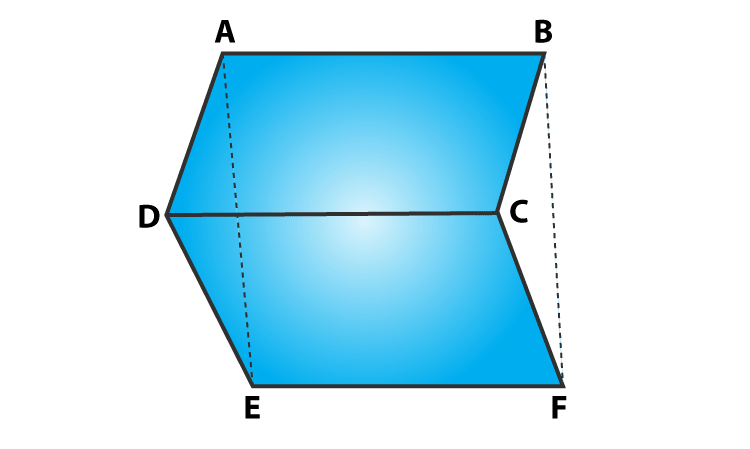Solution:

Here, ABCD, CDEF and ABFE are parallelograms:

Which implies:

DE = CF and

AE = BF

Again, from triangles ADE and BCF:

AD = BC, DE = CF and AE = BF

By SSS criterion of congruence, we have

Since both the triangles are congruent, then ar(ΔADE) = ar(ΔBCF).

Hence Proved,

Question 9: Diagonals AC and BD of a quadrilateral ABCD intersect each other at P. Show that: ar(ΔAPB) x ar(ΔCPD) = ar(ΔAPD) x ar(ΔBPC).

Solution:

Consider: BQ and DR are two perpendiculars on AC.

To prove: ar(ΔAPB) x ar(ΔCPD) = ar(ΔAPD) x ar(ΔBPC).

Now,

L.H.S. = ar(ΔAPB) x ar(ΔCDP)

= (1/2 x AP × BQ) × (1/2 × PC × DR)

= (1/2 x PC × BQ) × (1/2 × AP × DR)

= ar(ΔAPD) x ar(ΔBPC)

= R.H.S.

Hence proved.

Question 10: In figure, ABC and ABD are two triangles on the base AB. If line segment CD is bisected by AB at O, show that ar(ΔABC) = ar(ΔABD).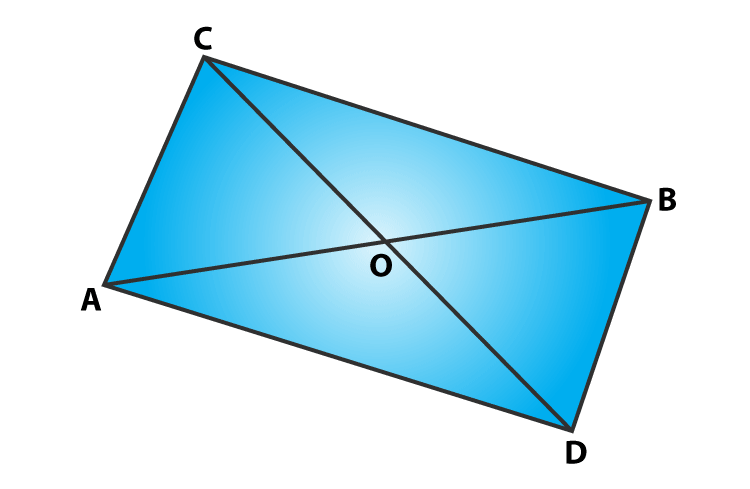Solution:

Draw two perpendiculars CP and DQ on AB.

Now,

ar(ΔABC) = 1/2×AB×CP ⋅⋅⋅⋅⋅⋅⋅(1)

ar(ΔABD) = 1/2×AB×DQ ⋅⋅⋅⋅⋅⋅⋅(2)

To prove the result, ar(ΔABC) = ar(ΔABD), we have to show that CP = DQ.

In right angled triangles, ΔCPO and ΔDQO

∠CPO = ∠DQO = 90o

CO = OD (Given)

∠COP = ∠DOQ (Vertically opposite angles)

By AAS condition: ΔCP0 ≅ ΔDQO

So, CP = DQ …………..(3)

(By CPCT)

From equations (1), (2) and (3), we have

ar(ΔABC) = ar(ΔABD)

Hence proved.

## RD Sharma Solutions for Class 9 Maths Chapter 15 Area of Parallelograms and Triangles Exercise 15.3

RD Sharma Solutions Class 9 Maths Chapter 15 Area of Parallelograms and Triangles Exercise 15.3 is based on the topic – Parallelogram on the same base and between the same parallels. In this exercise, students will learn how to compute the area of any quadrilateral which contains triangles.